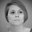## Deposit Products with Simple Interest

What: Add an option to set up deposit accounts with “Simple Interest” (and rename current method to “Compounded Interest”). If both simple and compounded interest methods are supported:
(a) Compounded Interest: current behaviour, i.e. after each Interest Applied transaction, interest for the next period is calculated based on previous balance + interest applied amount.
(b) Simple Interest: interest for each period is calculated only on the previous balance (either average or minimum, depending on the product specification).

For example, with a 5 year fixed deposit of 1,000,000 and yearly interest of 5%, each year interest in the amount 50,000 is applied. On the accounting side: Interest Expense would be debited and Interest Payable would be credited.

•Dorota Nosal